# Rational number

(diff) ← Older revision | Latest revision (diff) | Newer revision → (diff)

A number expressible as a fraction of integers. The formal theory of rational numbers is developed using pairs of integers. One considers ordered pairsof integersandfor which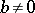. Two such pairs,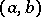and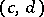, are called equivalent (equal) if and only if. This is an equivalence relation, being reflexive, symmetric and transitive, and so partitions the set of all such pairs into equivalence classes. A rational number is defined as an equivalence class of pairs. A pairis also called a rational fraction (or fraction of integers). Distinct classes define distinct rational numbers. The set of all rational numbers is countable. The rational number containing a pair of the form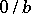is called zero. Ifis a rational number and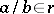, then the rational number containing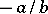is called the (additive) inverse of, and is denoted by. A rational numberis called positive (negative) if it contains a rational fraction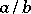withandof the same sign (of different signs). If a rational number is positive (negative), then its (additive) inverse is negative (positive). The set of rational numbers can be ordered in the following way: Every negative rational number is less then every positive one, and a positive rational number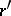is less than another positive rational number(written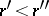) if there exist rational fractionsand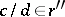,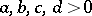, such that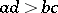; every negative (positive) rational numberis smaller (greater) then zero:(); a negative rational numberis less than another negative rational number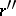if the positive rational number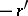is greater than the positive rational number: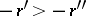. The absolute valueof a rational numberis defined in the usual way: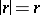if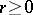andif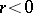.

The sum of two rational fractionsand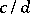is defined as the rational fraction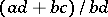and the product as. The sum and product of two rational numbersandare defined as the equivalence classes of rational fractions containing the sum and product of two rational fractionsandbelonging toand, respectively. The order, sum and product of rational numbersanddo not depend on the choice of representatives of the corresponding equivalence classes, that is, they are uniquely determined byandthemselves. The rational numbers form an ordered field, denoted by.

A rational numberis denoted by any rational fractionfrom its equivalence class, i.e.. Thus, one and the same rational number can be written as distinct, but equivalent, rational fractions.

If every rational number containing a rational fraction of the formis associated with the integer, then one obtains an isomorphism from the set of such rational numbers onto the ringof integers. Therefore, the rational number containing a rational fraction of the formis denoted by.

Each function of the form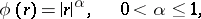(1)

is a norm on the field of rational numbers, that is, it satisfies the conditions:

1)for any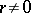,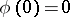;

2);

3)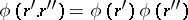for all. The field of rational numbers is not complete with respect to the norm (1). The completion ofwith respect to the norm (1) yields the field of real numbers.

Consider the function(2)

whereis a prime number,is a rational number andis determined by: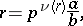where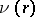is an integer,is an irreducible rational fraction such thatandare not divisible by, andis a fixed number,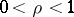. Then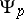is a norm on. It induces the so-called-adic metric.is not complete with respect to this metric. By completingwith respect to the norm (2), one obtains the field of-adic numbers (cf.-adic number). The metrics induced by (1) and (2) (for all prime numbers) exhaust all non-trivial metrics on.

In decimal notation, only rational numbers are representable as periodic decimals fractions.

How to Cite This Entry:
Rational number. Encyclopedia of Mathematics. URL: http://encyclopediaofmath.org/index.php?title=Rational_number&oldid=14864
This article was adapted from an original article by L.D. Kudryavtsev (originator), which appeared in Encyclopedia of Mathematics - ISBN 1402006098. See original article